www.derf.net / knapsack /

# NP-Completeness, Cryptology, and Knapsacks

## The Knapsack Problem

One day, our friend Bob is taken to a room full of toys and told that he can keep as many toys as he can fit in his knapsack (backpack). Clearly, he has to be careful -- he wants to be sure to get as many of the most fun toys as possible, without wasting space in his knapsack on the less fun toys. Being the clever guy that he is, he decides to assign a value (in terms of Fun-ness) to each toy. Then he figures out how much space each toy will take up in his knapsack. His knapsack is very flexible, so he doesn't have to worry about the shapes of the toys, only the sizes.

There are n toys in the room, so Bob constructs the n-component vectors f and s, such that the i-th component of f is the Fun value of the i-th toy, and the i-th component of s is the space that the i-th toy takes up. The total space Bob's knapsack can hold is K.

Now, choosing which toys to take amounts to finding a vector a of zeroes and ones such that S is no more than K, and F is maximal, where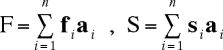(This of course is simply taking the dot products a . f and a . s.)

In a variation of the problem, Bob doesn't care so much about getting the absolute maximum Fun, he just wants to get F to be at least some Fun-ness goal G.

[back to ToC]

## An Overview of NP and NP-Completeness

The term "NP" refers to a class of problems which could be solved by a nondeterministic computer in an amount of time which varies as some polynomial in the size of the input (hence NP, for Nondeterministic Polynomial time). Nondeterminism is a theoretical model of computation wherein the computer is allowed to "guess" what to do, and if there is a set of guesses which leads the computer to an answer, then the computer is assumed to guess correctly; if there is no correct set of guesses, then the computer will keep trying forever. This concept of a guessing computer is difficult to grasp at first, since it is purely theoretical and not in fact implementable.

Of course, any computation that can be performed by a deterministic computer can also be done by a nondeterministic computer, simply by never having to guess anything. So therefore everything in P, the class of problems with deterministic polynomial solutions, lies within NP. Although a deterministic computer could simulate a nondeterministic computation (by trying all possible guesses, for instance), the time spent will clearly be much greater. It is not known whether P=NP, though it is widely believed that there are problems in NP which are not in P. Some such problems have a property known as NP-completeness. These are problems which are in NP and which are as "hard" as any other problem in NP. What we mean by "hard" is of course the crux of the meaning of NP-completeness, as will become more clear after an example.

The canonical example of an NP-complete problem is the SAT problem (that's "Sat.", not "S.A.T."). This is the question of satisfiability of formulas in propositional logic. Given a formula, such as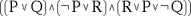we ask the question, "is there a set of assignments (true or false) to the variables in the formula (P, Q, R) which makes the formula evaluate to true?" In this simple instance, it's not hard to find such an assignment -- the assignment "P=F, Q=T, R=T" works, for example. But in general, if the formula is much larger, it is very hard to tell. If you had some way of guessing the assignment, then it is trivial to verify that indeed the formula is satisfied. This is precisely the idea behind nondeterminism. But if there is no satisfying assignment to a particular formula, you might have to list all possible assignments (of which there are an exponential number), and try out each one to see that in fact it falsifies the formula. This property of a "YES" answer being easy to verify but a "NO" answer taking much longer is typical of NP-complete problems.

We won't go into the proof, but it turns out that if you came up with a way to solve SAT in (deterministic) polynomial time, then every problem in NP could be solved in polynomial time, using that polynomial SAT algorithm as a subroutine. Thus no other NP problem is "harder" to solve than SAT -- this is the sense in which we meant "hard" above.

Now suppose you take another NP problem -- call it X -- and show that solving X in polynomial time allows you to solve SAT in polynomial time. Then since we can use a polynomial SAT algorithm to solve any NP problem, we can also use a polynomial X algorithm to solve any NP problem; so X is also NP-complete. This is an NP-completeness reduction. NP-completeness reductions are confusing at first, as they seem somewhat backwards.

If X is known to be NP-complete, then you can reduce another NP problem Y to X, and then Y is NP-complete: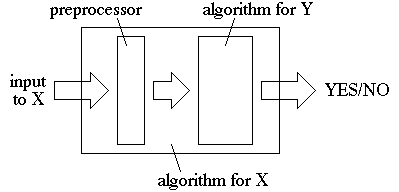Note that the output of the preprocessor must be a valid input to Y. Now if the preprocessor is polynomial time, then if Y were in P, X would also be in P. And if X is NP-complete then its membership in P implies all problems in NP are in P, i.e. P=NP. So then Y must be NP-complete as well. Again, these reductions always seem backwards until you get used to the concept.

To show that Y is NP-complete from knowledge that X is NP-complete we have to describe the preprocessor algorithm, but the algorithm for Y (the box on the right in the above diagram) is purely hypothetical. And the proof then must show each of the following: that Y is in NP, that the preprocessor is in polynomial time, and that the algorithm for Y when run on the instance outputted by the preprocessor will say "YES" if and only if the answer for the original instance of X should be "YES".

[back to ToC]

## An Overview of Public Key Cryptography

The idea behind public key cryptography is fairly simple: Anyone can put something in a box and close the lock, but only the person who knows the lock combination can open the box again. More literally, suppose Alice has a public key, which she publishes. Then anyone can encrypt a message to send her. And everyone uses the same method of encryption. But only Alice knows the secret key information which allows her to invert the process and decrypt the message.

In general, these systems rely on the intractability of computing some function, or, more precisely, some function's inverse. For instance, many systems are based on the fact that it is computationally infeasible to compute discrete logarithms. Suppose Alice raises 2 to the power of some number x, in the integers mod p, where p is a very large prime, and she tells Bob the result and p. Then there is probably no way for Bob to figure out what x was in a reasonable amount of time. Of course, he can just raise 2 to lots of numbers until he gets the same result, but in general, this will take a very long time. So, because it is very difficult to invert modular exponentiation, we call it a one-way function. (We don't have to use 2, we can use any primitive element of the integers mod p.)

However, a one-way function isn't terribly useful for encryption purposes, since even the person intended to be able to decipher the message cannot. So instead, to be useful for encrypting, we need to set up a one-way trapdoor function -- a function which, although it is difficult to invert, becomes easy to invert when you know some special information (the secret key).

At the time that the idea of a trapdoor one-way function was proposed, the above one-way function was known, but no trapdoor function was actually known to exist. Indeed, the trapdoor functions that are now used are still not in fact proven to be one-way, though they are fairly widely believed to be.

[back to ToC]

## The NP-Completeness of Knapsack

Knapsack is certainly in NP, since we can guess which toys to take (i.e. which bits of a to make 1 and which 0), and then it only takes a polynomial number of steps to check whether we met the goal G.

We will reduce the Exact Cover by 3-Sets (EC3S) problem to Knapsack. EC3S is quite simple to state, and it's known to be NP-complete. Suppose we haveIn other words, we have a collection of n sets, each of which is a subset of a certain set U, and each of which has three elements. Furthermore, U has 3m elements, where m is some positive integer.

Now, EC3S asks whether there is a subset of A which forms a partition of U. In other words, whether there is a set B, such that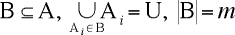So now let us perform the reduction. We are given an instance of EC3S, from which we construct an instance of Knapsack. As it turns out, we construct a very special case of Knapsack, where f = s, and G = K. So we actually show that this special case is NP-complete, but then the more general case must be NP-complete as well (since it can't be any easier than its special case).

So we have A, in the form of n vectors, each of which has m components; further, each vector has exactly three components which are 1's, and the rest are 0's. If we think of these m-component vectors as m-bit binary numbers, then adding the numbers corresponds exactly to taking the union of the sets, except for the "carrying". So to ensure that we never carry, we think of the vectors as numbers in base n+1, rather than base 2. And a set of sets which form an exact cover corresponds to a set of numbers whose sum is the number "111...1" (m 1's), also a number in base n+1.

So we will choose f and s so that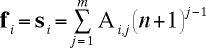In other words, we simply let f hold the numbers that correspond to the vectors of A. And we choose both the goal G and the size of the knapsack K to be "111...1" (base n+1). Now we have constructed our instance of Knapsack.

If we solve this Knapsack instance the solution to the corresponding EC3S problem is obvious -- there will be an exact cover if and only if there is a set which fills the knapsack.

[back to ToC]

## The Knapsack One-Way Function

In the special case of Knapsack used for the reduction above, we are given f and K, and asked to find a bit vector x so that f . x = K. Since this question is NP-complete, there is no efficient algorithm known to compute x from f and K, in general. So we can use a vector f to encipher an n-bit message x, by just taking K = f . x.

It is important to note that the choice of f is crucial. For instance, suppose that f is chosen to be a superincreasing sequence. This is the situation where for each i,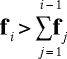In this case, given f and K, it is simple to compute x. We can just check whether K is larger than the last element of f; if it is, we make the last element of x a 1, subtract that value from K, and then recursively solve the smaller problem. This works because when K is larger than the last element of f, even if we choose x = (1 1 1 ... 1 0), the dot product f . x will be too small, because of the superincreasing property. So we must choose a 1 in the last position of x.

Clearly the choice of f is important -- depending on f, we may or may not have a one-way function. However, the existence of this easy case is exactly what allows us to create the Knapsack trapdoor one-way function, which we can use for a public-key cryptosystem.

[back to ToC]

## The Merkle-Hellman Knapsack Public Key Cryptosystem

Alice generates her public key as follows:

1. Generate a superincreasing sequence f ', with about 100 components.
2. Choose a random integer m > the sum of the elements of f '.
3. Choose another random integer w relatively prime to m.
4. Now generate f '' by multiplying each component of f ' by w mod m: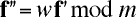5. And finally permute f '' by a random permutation matrix P to obtain the public key f.

Now Alice makes f public, and keeps f ', m, w, and P secret.

When Bob wants to send Alice a message (bit vector) x, he computes S = f . x, and sends this S. If this system is secure, then to the outside observer Eve (the evil eavesdropper), finding x from S and the public key f should amount to solving a generic instance of Knapsack, which is NP-complete. For the moment let us assume this is true. Then although Eve cannot decrypt the message, Alice can, using the secret values she used to generate f.

Alice can find the number S ' = f ' . x, so that she can solve the associated easy Knapsack problem with the superincreasing f '. To see how to compute S ', consider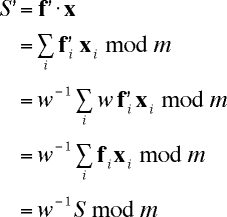So Alice just multiplies S by the inverse of w mod m and then solves the superincreasing knapsack problem f ', S ', and now she can read her message.

When we generate a key, we start with a superincreasing sequence, and then (apparently) hide the structure that is present in the sequence. In other words, the sequence that is constructed seems not to have any structure to it. But the system can be made even stronger (or so it would seem), by iterating this process of hiding structure. If we choose another m and w, we can generate a new public key, which, it turns out, cannot be generated using any single (m,w) pair. We can do this many times, and it seems that the system becomes more and more secure with each iteration.

[back to ToC]

## Attacks on Knapsack Systems

When Ralph Merkle proposed the above system in 1976, he was certain it was secure, even in the single-iteration case, and offered \$100 to anyone who could break it.

In 1982, Adi Shamir discovered an attack on single-iteration knapsack systems. It was somewhat narrow, and soon after there were ways announced to modify the original scheme which then prevented the attack. Nonetheless, Merkle paid the \$100 as promised, and more importantly, this very important breakthrough was the beginning of the fall of knapsack systems.

For the moment, let us assume that no permutation is used, so f = f ''. Then for each i, we have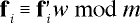So by definition of modular congruence, there must exist a vector k, such that for each i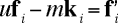where u is the multiplicative inverse of w in the integers mod m (remember we chose w to be relatively prime to m, so this inverse does exist). Then, dividing gives us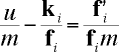Since m is very large, the expression on the right is very small, so each element of the component-wise quotient of k and f is close to u / m. Plugging in 1 for i and subtracting from the original equation, we getSince both quantities on the right hand side are positive, and the quantity being subtracted is very small, we can say,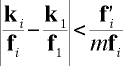Also note that because f ' is superincreasing, each element must be less than half its successor, so for each i,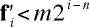Then we can also say that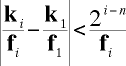Rearranging this we get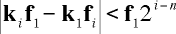It turns out that since f is public, only a few of these inequalities (three or four of them) uniquely determine k. These inequalities are an instance of integer programming, so the Lenstra integer linear programming algorithm will find k quickly. And once we know k, it is easy to break the system.

Suppose we do permute f before publishing it, i.e. P is not the identity. Since we only need the first 3 or 4 elements of k, we can just try all possibilities, of which there are only a cubic or quartic number.

Even after paying Shamir the \$100, Merkle was certain that a multiple-iterated system was secure, and he offered \$1000 to anyone who could break that. It took two years, but in the summer of 1984, Merkle had to pay the one grand to Ernie Brickell, who had broken a system of 40 iterations in about an hour of Cray-1 time.

Attacks have been developed for more general knapsack systems, and now any system which uses modular multiplication to disguise an easy-to-solve knapsack can be broken efficiently. However, as we shall see, this is not the only way to use knapsacks in cryptography.

[back to ToC]

## The Chor-Rivest Knapsack Cryptosystem -- A Different Approach

In 1986, Ben-Zion Chor came up with this knapsack system which is the only one yet which does not rely on modular multiplication to disguise an easy knapsack. This is also the only knapsack system which has not been broken.

First, note that any superincreasing sequence must grow exponentially fast, since the minimal superincreasing sequence is the powers of 2. Second, note that the reason a superincreasing sequence is useful is that every h-fold sum from the sequence is unique. In other words, if we represent our sequence as a vector f, then the function of "dotting" f with a bit vector x is one-to-one, and so it can be inverted. But it is possible to have a sequence which grows only polynomially fast and which still has the property of uniqueness of h-fold sums. The question of whether such a sequence exists was posed in 1936; in 1962, R. C. Bose and S. Chowla published the following construction for such a sequence.

Some notation: Let GF(p) be the field of integers modulo p, and let GF(p,h) be the h-degree extension field of the base field GF(p), i.e. GF(p,h) is the field obtained by adjoining an element of degree h to GF(p). (GF(p,h) is often noted with a superscript h, since the number of elements in the field is p raised to the power h.) Also, let 1 be the vector in which each entry is a "1".

Formally, what we are constructing is a sequence a of length p such that for i from 0 to p - 1,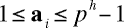and for any distinct x, y, with x . 1 = y . 1 = h, x . a and y . a should also be distinct. Although it is not necessary for the construction, we can think of x and y as bit vectors (i.e. containing only 0's and 1's); we should require, however, that they contain only nonnegative values.

Now the construction is fairly simple. First, take t, algebraic of degree h over GF(p); i.e. the minimal polynomial with coefficients in GF(p) with t as a root is of degree h. Next take g, a multiplicative generator (primitive element) of GF(p,h); i.e. for each element x of GF(p,h) (besides zero), there is some number i such that g raised to the power i is x.

Now consider an additive shift of GF(p), i.e. the set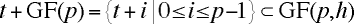Let each element of a be the logarithm to the base g of the corresponding element of t + GF(p):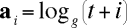We must verify that a defined this way satisfies the desired properties. Certainly each element of a will be in the desired range, since g generates GF(p,h). Now suppose that we have distinct x and y, with x . 1 = y . 1 = h, but x . a = y . a. Then raising g to the power of x . a and y . a, we have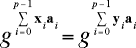So we can also say,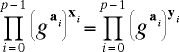And then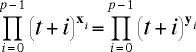Now, notice that the product on each side of the equation is a monic polynomial in t of degree h. In other words, if we were to multiply these two products out, and replace the value t with a formal parameter, say x, then the highest term on each side would be x raised to the power h, with a coefficient of 1. We know that if we plug in the value t for x, the values of the two polynomials will be equal. So we subtract one polynomial from the other, the highest terms cancel out, and if we plug in t, we get 0. This gives us a polynomial of degree h - 1, with t as a root. But this contradicts the fact that we chose t to be algebraic of degree h. So the proof is complete, and the construction is valid.

Chor developed a method for using this construction as the basis of a cryptosystem. Basically, we choose p and h small enough so that we will be able to compute some discrete logs in GF(p,h). Chor recommends p around 200 and h around 25. Then, we pick t and g as above. There will be many possibilities to choose from for each of these, and we can simply pick randomly among them. (In fact, there will be so many t,g pairs that a very large number of users can use the same p and h, and the probability of two people generating the same key is negligible.) Then we follow the Bose-Chowla construction: We compute the base g logarithms of t + i for each i, and that gives us a. Finally, we choose a random permutation of a, and that is our key. We publish the permuted a, along with p and h. We keep secret t, g, and the permutation that was used.

To send a message to Alice, Bob simply takes his message x and computes x . a = s. Actually, it's not quite this simple, since the message must be p bits long and must have x . 1 = h, but Chor provides a fairly straightforward method for transforming an unconstrained bit string to several blocks, each having the required form. Alice receives s. She raises g to the power of s and expresses the result as a polynomial in t of degree h, with coefficients in GF(p). She computes the h roots of this polynomial by successive substitutions. Then she applies the inverse permutation to the values of the roots, and attains the indices of x which hold the bit 1.

It is interesting to note that if anyone ever discovers an efficient method for computing discrete logs, not only will that algorithm not help to break this system, but also, since we have to take some small discrete logs to generate a key, key generation will probably be made easier.

A number of attacks on this system have been published using knowledge of some part of the secret key, but there has not yet been any attack shown to break this system efficiently knowing only the public key.

[back to ToC]

## References

• Chor, Ben-Zion. Two Issues in Public Key Cryptography. Cambridge: MIT Press, 1986.